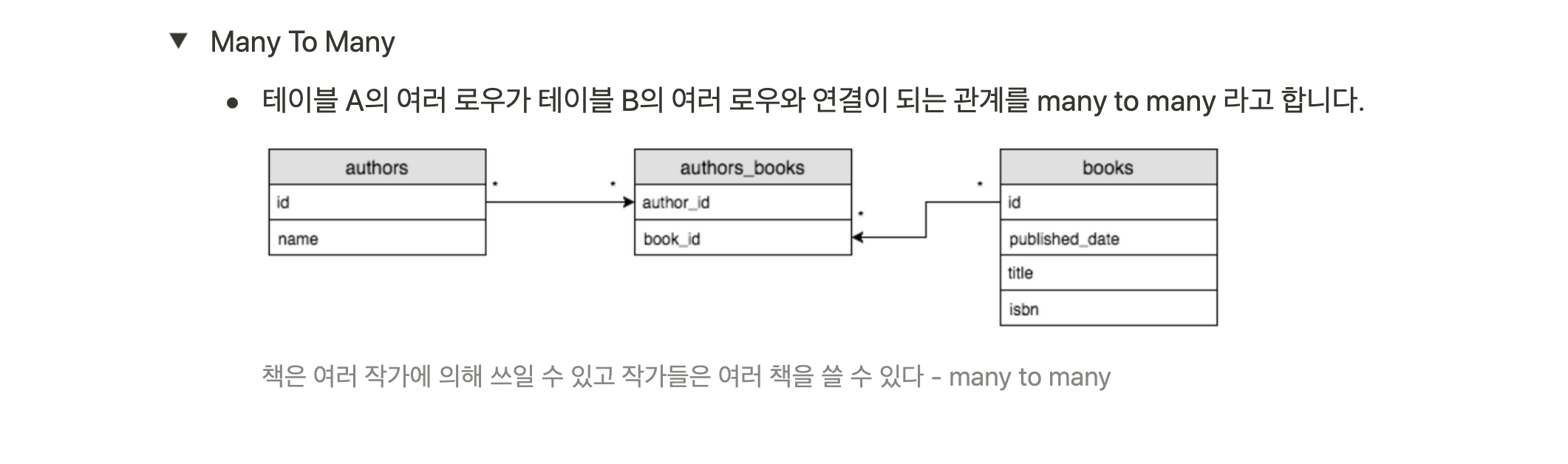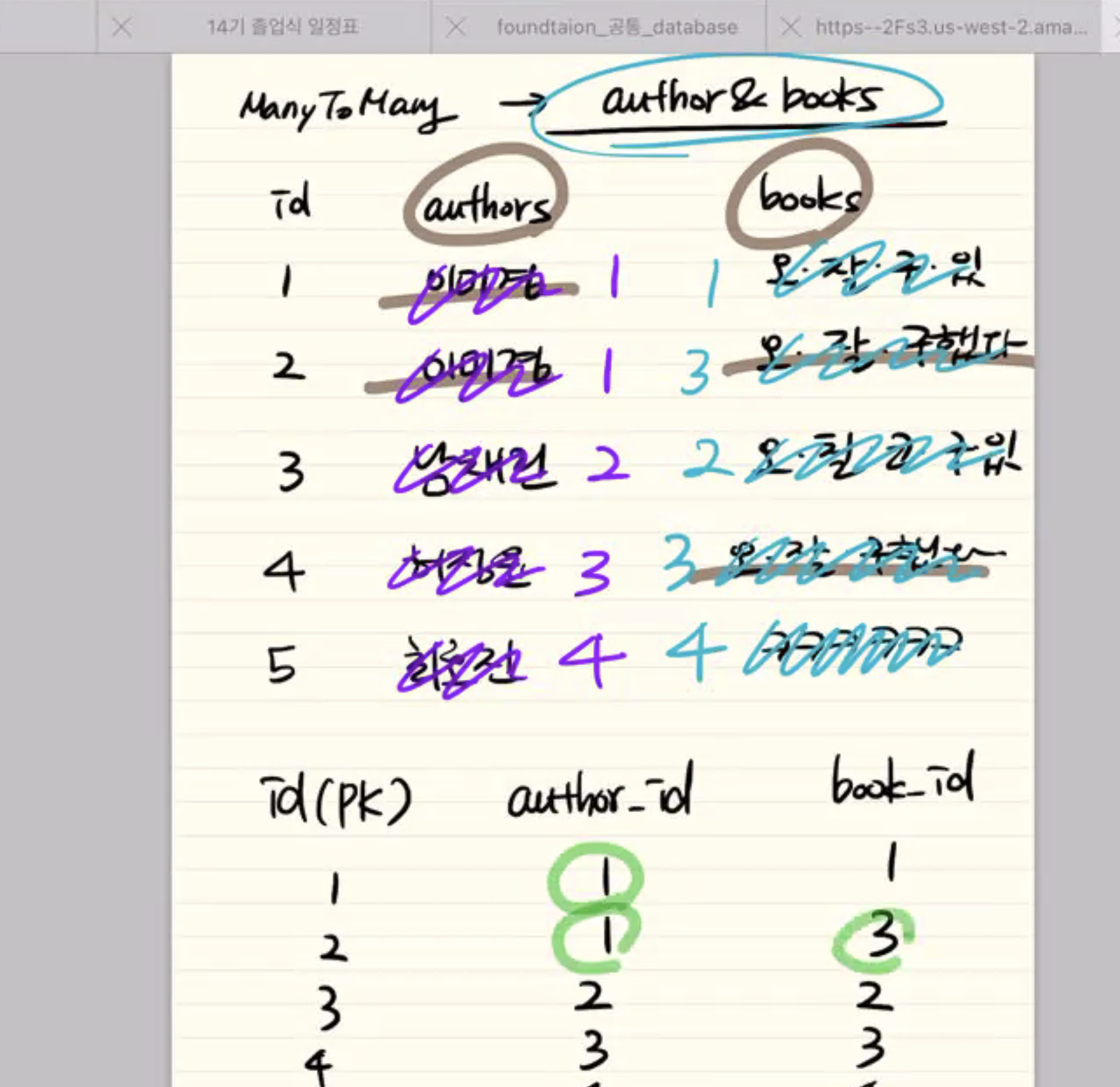# TIL: Databases

markkimjr·2021년 1월 19일
0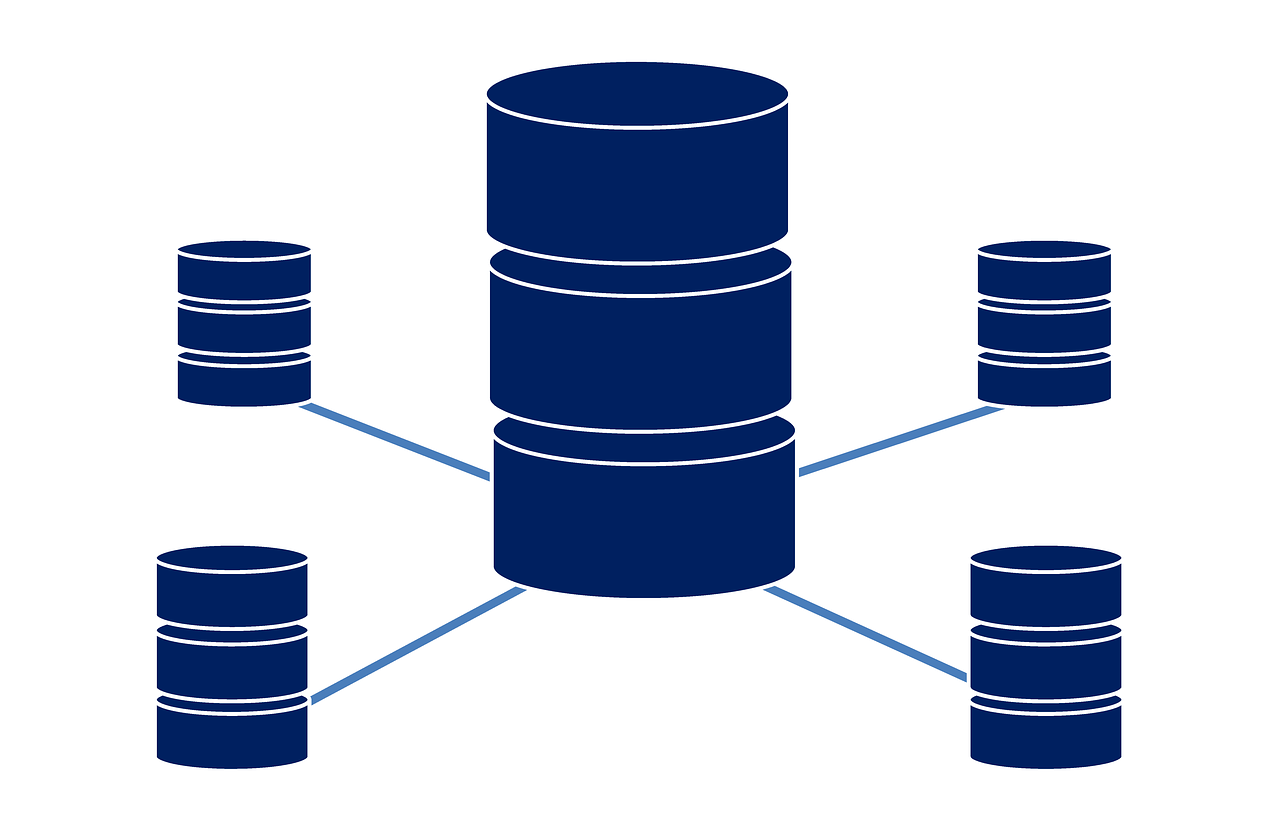# Database

Where a computer system's data is stored

• Two main types of databases:
1. Relational databases (RDBMS)
2. Non-relational databases (NoSQL)

## Relational Databases

Data is stored and divided into different tables, which are related to each other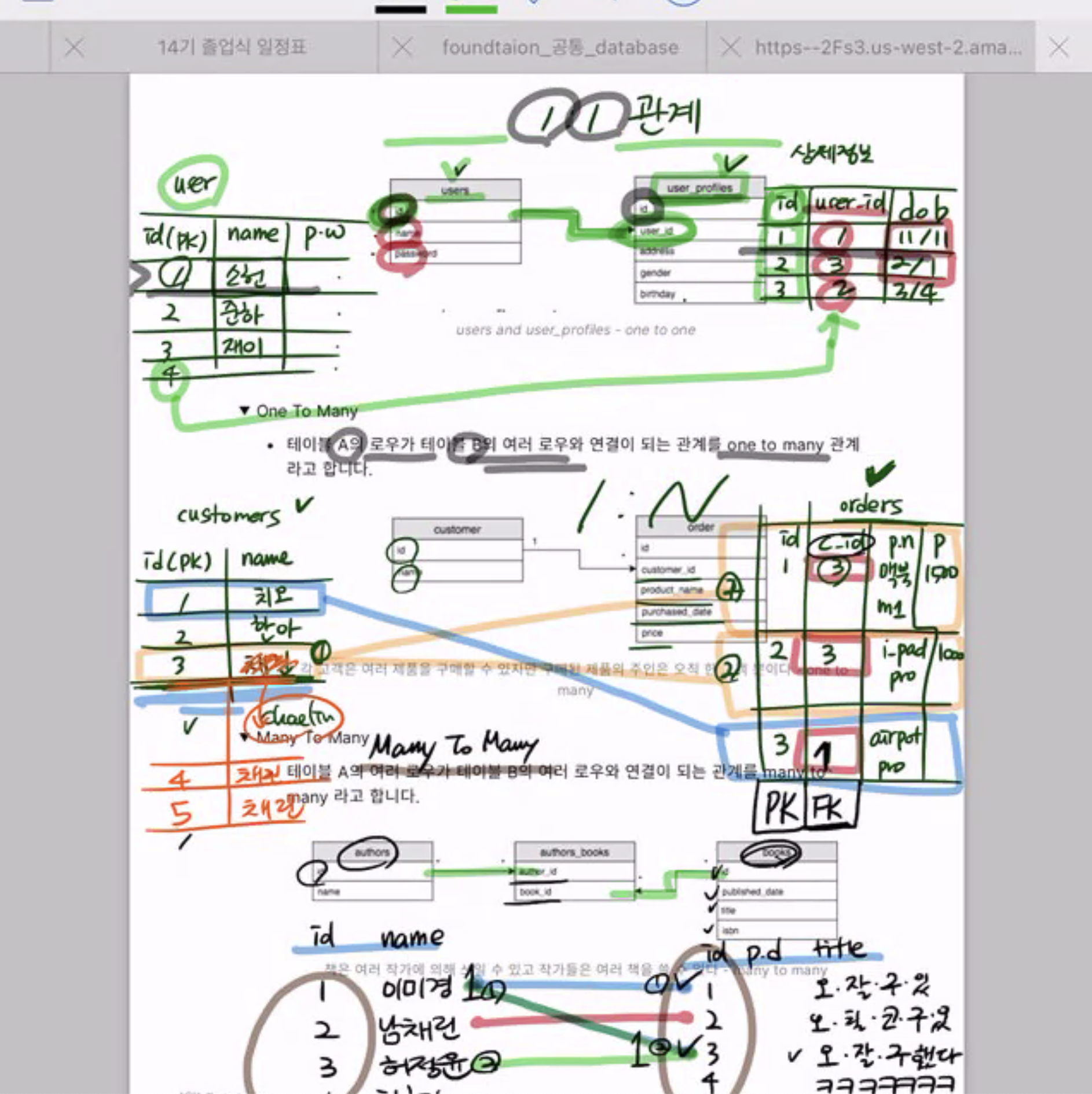### One to One

When rows between 2 tasbles match exactly one to one
i.e. Table A (students) and Table B (student key cards) --> Each row of students matches one-to-one with each row of student key cards. Table B's user_id is the foreign key (FK) that connects it to table A (table A's primary key)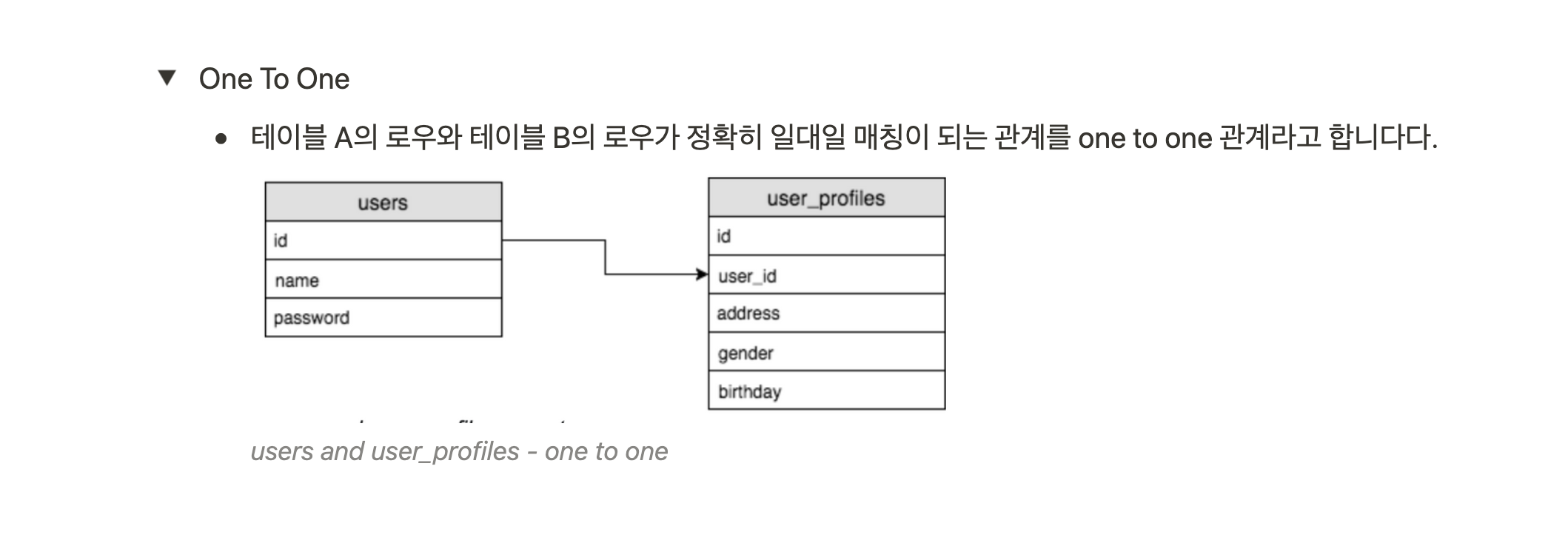### One to Many

When a row from one table connects to many rows in another table
i.e. Table A (customers) and Table B (customer orders) Each customer can place many customer orders, so one row from table A can match with many rows from Table B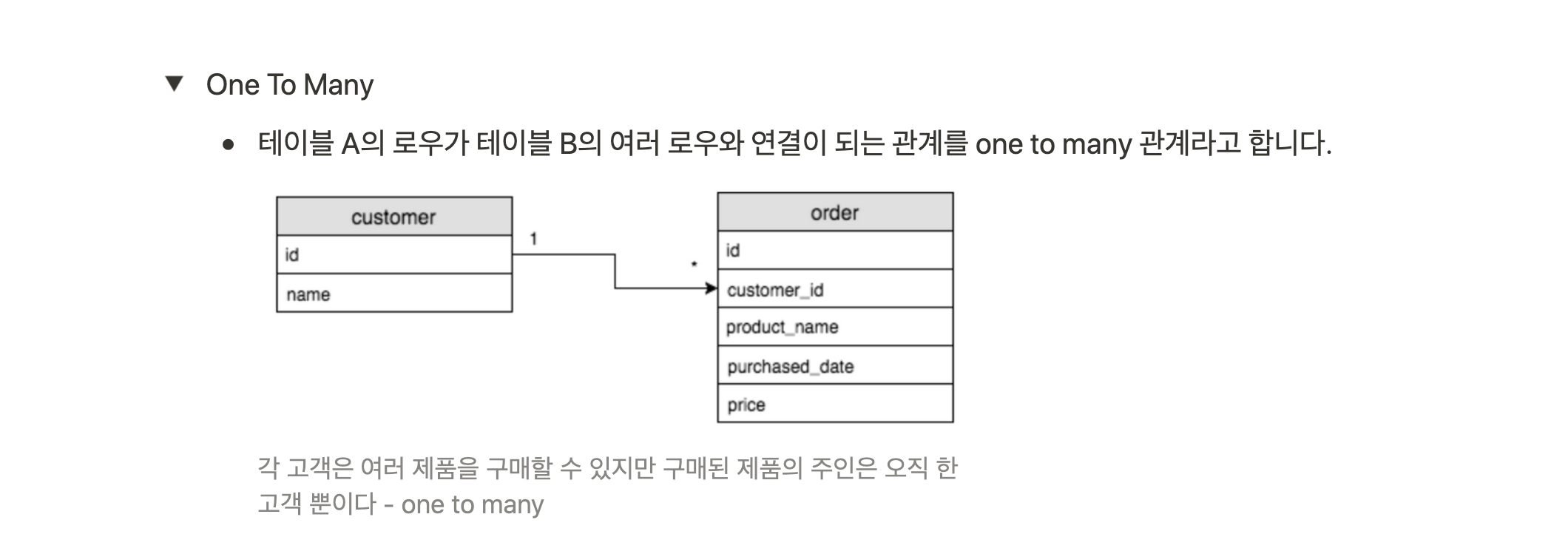### Many to Many

When rows from one table connect to many rows in another table and vice versa
i.e. Table A (authors) and Table B (books). One author can write many books and books with the same title can have many authors (there can be more than one book with the same title). In this case, there must be a connector table that connects Table A and Table B